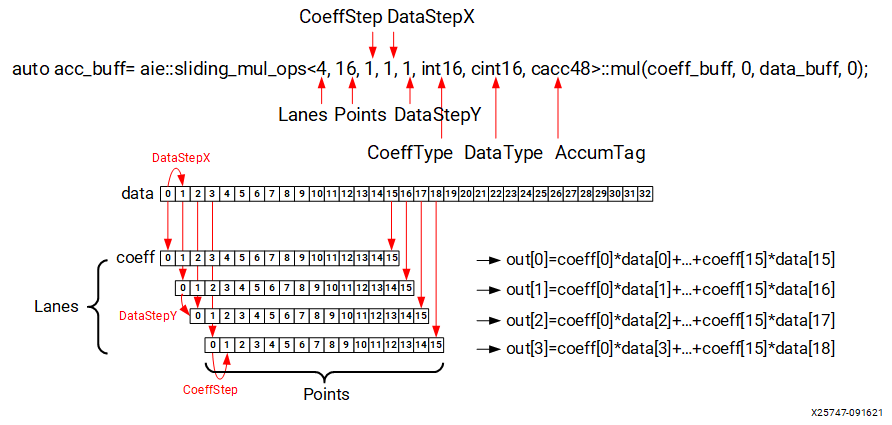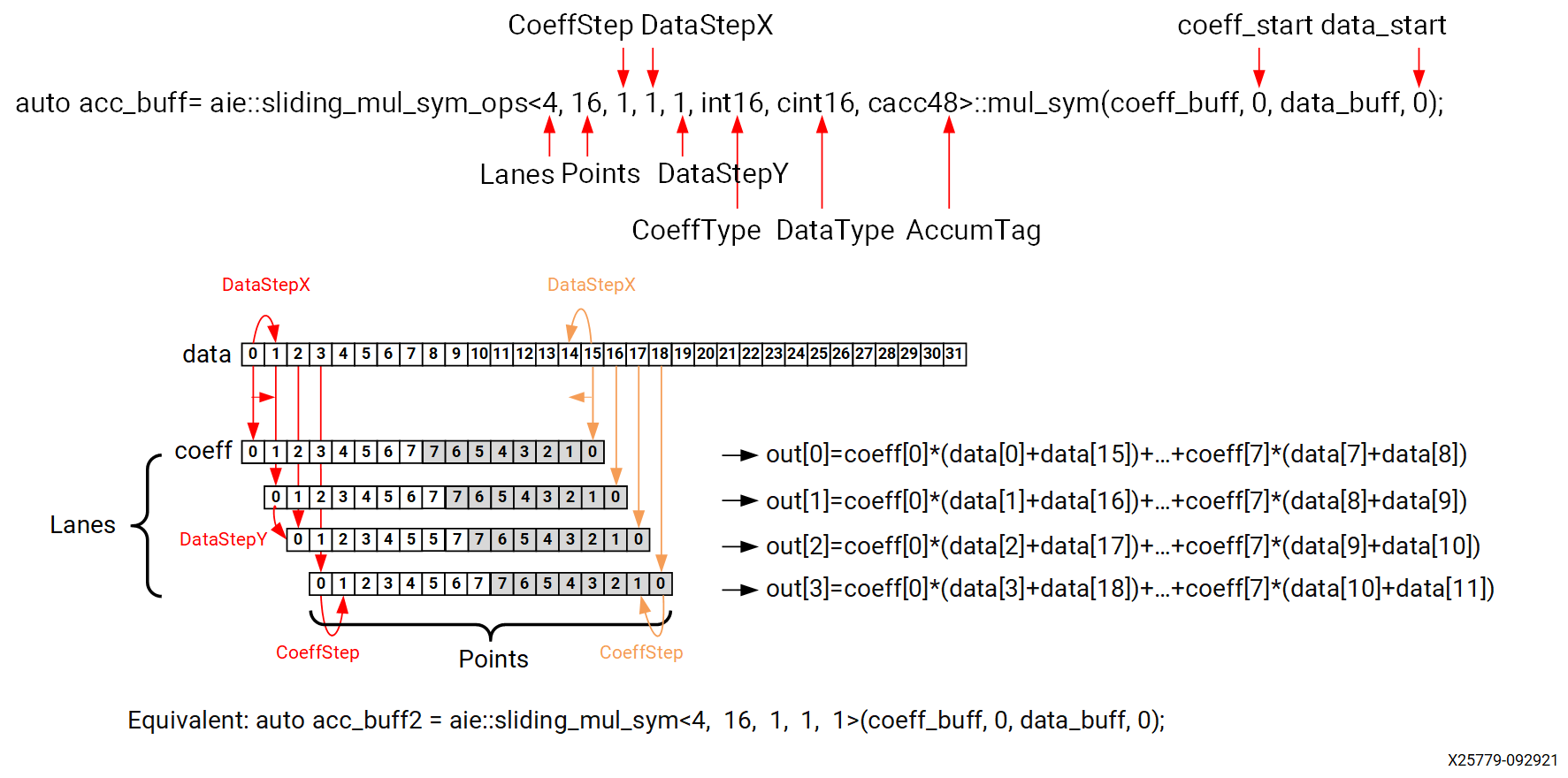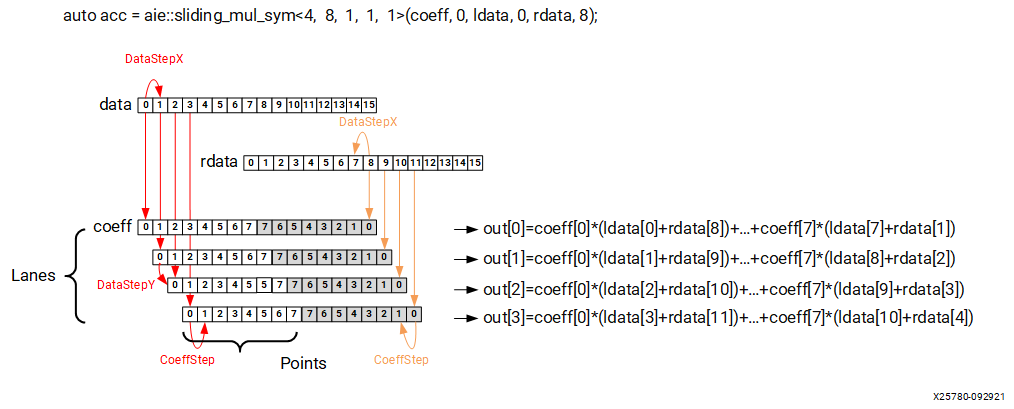# Multiple Lanes Multiplications - sliding_mul - 2022.2 English

## AI Engine Kernel and Graph Programming Guide (UG1079)

Document ID
UG1079
Release Date
2022-10-19
Version
2022.2 English

AI Engine provides hardware support to accelerate a type of multiple lanes multiplications, called sliding multiplication. It allows multiple lanes to do MAC operations simultaneously, and the results are added to an accumulator. It especially works well with (but not limited to) finite impulse response (FIR) filter implementations.

These special multiplication structures or APIs are named `aie::sliding_mul*`. They accept coefficient and data inputs. Some variants of `aie::sliding_mul_sym*` allow pre-adding of the data input symmetrically before multiplication. These classes include:

• `aie::sliding_mul_ops`
• `aie::sliding_mul_x_ops`
• `aie::sliding_mul_y_ops`
• `aie::sliding_mul_xy_ops`
• `aie::sliding_mul_sym_ops`
• `aie::sliding_mul_sym_x_ops`
• `aie::sliding_mul_sym_y_ops`
• `aie::sliding_mul_sym_xy_ops`
• `aie::sliding_mul_sym_uct_ops`

For more information about these APIs and supported parameters, see the AI Engine API User Guide (UG1529).

For example, the `aie::sliding_mul_ops` class provides a parametrized multiplication that implements the following compute pattern.

``````DSX = DataStepX
DSY = DataStepY
CS = CoeffStep
P = Points
L = Lanes
c_s = coeff_start
d_s = data_start
out = coeff[c_s] * data[d_s + 0] + coeff[c_s + CS] * data[d_s + DSX] + ... + coeff[c_s + (P-1) * CS] * data[d_s + (P-1) * DSX]
out = coeff[c_s] * data[d_s + DSY] + coeff[c_s + CS] * data[d_s + DSY + DSX] + ... + coeff[c_s + (P-1) * CS] * data[d_s + DSY + (P-1) * DSX]
...
out[L-1] = coeff[c_s] * data[d_s + (L-1) * DSY] + coeff[c_s + CS] * data[d_s + (L-1) * DSY + DSX] + ... + coeff[c_s + (P-1) * CS] * data[d_s + (L-1) * DSY + (P-1) * DSX]``````
Table 1. Template Parameters
Parameter Description
Lanes Number of output elements.
Points Number of data elements used to compute each lane.
CoeffStep Step used to select elements from the coeff register. This step is applied to element selection within a lane.
DataStepX Step used to select elements from the data register. This step is applied to element selection within a lane.
DataStepY Step used to select elements from the data register. This step is applied to element selection across lanes.
CoeffType Coefficient element type.
DataType Data element type.
AccumTag Accumulator tag that specifies the required accumulation bits. The class must be compatible with the result of the multiplication of the coefficient and data types (real/complex).

The following figure shows how to use the `aie::sliding_mul_ops` class and its member function, `mul`, to perform the sliding multiplication. It also shows how each parameter corresponds to the multiplication.

Figure 1. sliding_mul_ops Usage ExampleBesides the `aie::sliding_mul*` classes, AI Engine API provides `aie::sliding_mul*` functions to do sliding multiplication and `aie::sliding_mac*` functions to do sliding multiplication and accumulation. These functions are simply helpers, that use the `aie::sliding_mul*_ops` classes internally and are provided for convenience. These include:

• `aie::sliding_mul`
• `aie::sliding_mac`
• `aie::sliding_mul_sym`
• `aie::sliding_mac_sym`
• `aie::sliding_mul_antisym`
• `aie::sliding_mac_antisym`
• `aie::sliding_mul_sym_uct`
• `aie::sliding_mac_sym_uct`
• `aie::sliding_mul_antisym_uct`
• `aie::sliding_mac_antisym_uct`

The following examples perform asymmetric sliding multiplications (template prototypes are in comments for quick reference).

``````/*template<unsigned Lanes, unsigned Points, int CoeffStep, int DataStepX, int DataStepY, ElemBaseType CoeffType, ElemBaseType DataType, AccumElemBaseType AccumTag = detail::default_accum_tag_t<CoeffType, DataType>>
struct aie::sliding_mul_ops< Lanes, Points, CoeffStep, DataStepX, DataStepY, CoeffType, DataType, AccumTag >
template<VectorOrOp VecCoeff, VectorOrOp VecData>
static constexpr accum_type mul (const VecCoeff &coeff, unsigned coeff_start, const VecData &data, unsigned data_start)
*/

aie::vector<int16,16> va;
aie::vector<int16,64> vb0,vb1;
aie::accum<acc48,8>  acc = aie::sliding_mul_ops<8, 8, 1, 1, 1, int16, int16, acc48>::mul(va, 0, vb0, 0);
acc = aie::sliding_mul_ops<8, 8, 1, 1, 1, int16, int16, acc48>::mac(acc, va, 8, vb1, 0);
window_writeincr(out,acc.to_vector<int32>(15));

/*template<unsigned Lanes, unsigned Points, int CoeffStep = 1, int DataStepX = 1, int DataStepY = DataStepX, AccumElemBaseType AccumTag = accauto, VectorOrOp VecCoeff = void, VectorOrOp VecData = void>
auto sliding_mul (const VecCoeff &coeff, unsigned coeff_start, const VecData &data, unsigned data_start)
*/

aie::vector<int32,32> data_buff;
aie::vector<int32,8> coeff_buff;
aie::accum<acc80,8> acc_buff = aie::sliding_mul<8, 8>(coeff_buff, 0, data_buff, 0);``````

Following are symmetric sliding multiplication examples.

``````/*template<unsigned Lanes, unsigned Points, int CoeffStep, int DataStepX, int DataStepY, ElemBaseType CoeffType, ElemBaseType DataType, AccumElemBaseType AccumTag = detail::default_accum_tag_t<CoeffType, DataType>>
struct aie::sliding_mul_sym_ops<Lanes, Points, CoeffStep, DataStepX, DataStepY, CoeffType, DataType, AccumTag >

template<VectorOrOp VecCoeff, VectorOrOp VecData>
static constexpr accum_type mul_sym (const VecCoeff &coeff, unsigned coeff_start, const VecData &data, unsigned data_start)
*/

aie::vector<cint16,16> data_buff;
aie::vector<int16,8> coeff_buff;
auto acc_buff = aie::sliding_mul_sym_ops<4, 16, 1, 1, 1, int16, cint16, cacc48>::mul_sym(coeff_buff, 0, data_buff, 0);//usage 1

/*template<unsigned Lanes, unsigned Points, int CoeffStep = 1, int DataStepX = 1, int DataStepY = DataStepX, AccumElemBaseType AccumTag = accauto, VectorOrOp VecCoeff = void, VectorOrOp VecData = void>
auto sliding_mul_sym (const VecCoeff &coeff, unsigned coeff_start, const VecData &data, unsigned data_start)
*/

auto acc_buff2 = aie::sliding_mul_sym<4, 16, 1, 1, 1>(coeff_buff, 0, data_buff, 0);//usage 2: equivalent to above

/*template<unsigned Lanes, unsigned Points, int CoeffStep = 1, int DataStepX = 1, int DataStepY = DataStepX, AccumElemBaseType AccumTag = accauto, VectorOrOp VecCoeff = void, VectorOrOp VecData = void>
auto sliding_mul_sym (const VecCoeff &coeff, unsigned coeff_start, const VecData &ldata, unsigned ldata_start, const VecData &rdata, unsigned rdata_start)
*/

aie::vector<cint16,16> ldata,rdata;
aie::vector<int16,8> coeff;
auto acc = aie::sliding_mul_sym<4, 8, 1, 1, 1>(coeff, 0, ldata, 0, rdata, 8);//symmetric sliding_mul using two data registers.``````
Note: All registers in sliding multiplication must be considered circular. They go back to the start after they reach the end.

The following figures show how the previous symmetric examples are computed.

Figure 2. sliding_mul_sym_ops Usage ExampleFigure 3. sliding_mul_sym Function with Two Data Registers## Considerations When Using sliding_mul

Some restrictions include:
• Data width <=1024 bits, and Coefficient width <=256bits
• Lanes * Points >= (MACs per cycle for that type)
• int8 is not supported